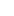# Tutor Hunt Questions

Question 13

Acorns limited produce and sells a single product known as the acres. The following details relate to the production of one unit of the acre.
\$ Per acre
Material cost = 12.00
Labour cost (1 hour) = 10.00

The budgeted and actual details for the month are as follows:

Budgeted production 6,000 acres
The selling price per acre is \$100
Actual production was 6,200 acres
Opening inventory 800 acres
Closing inventory 600 acres.

The actual fixed production overhead cost for the month is the same as the budgeted fixed cost at \$30,000. The company uses a predetermined overhead absorption rate calculated based on labour hours to absorb fixed overheads to products. The budgeted labour hours were 6,000.

The other overhead costs of the company are as follows:

Selling and distribution:
Fixed per month =\$10,000
Variable per unit =\$3

Fixed per month =\$5,000

Required:
a.
(i) Calculate the fixed production overhead cost per unit.
(3 Marks)
(ii) Calculate the number of units sold during the month.
(4 Marks)
(iii) Calculate the production cost per unit
(3 Marks)
(iv) Calculate the over/under absorbed overhead cost for the period.
(2 Marks)

b. Prepare a profit statement under absorption costing for the month of May.
4 years ago